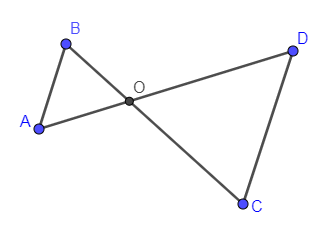Filters
Latest Questions
Mathematics
Vertically opposite angles
How do you identify vertical angles?
Mathematics
Vertically opposite angles
In the adjacent figure, \$\angle B< \angle A\$ and \$\angle C< \angle D\$ . Show that \$AD< BC\$.Mathematics
Vertically opposite angles
State true or false:
If two lines intersect and if one pair of vertically opposite angles is formed by acute angles, then the other pair of vertically opposite angles will be formed by obtuse angles.
(a) True
(b) False

Mathematics
Vertically opposite angles

What is the opposite of the following:

100m above sea level

Prev
1
Next